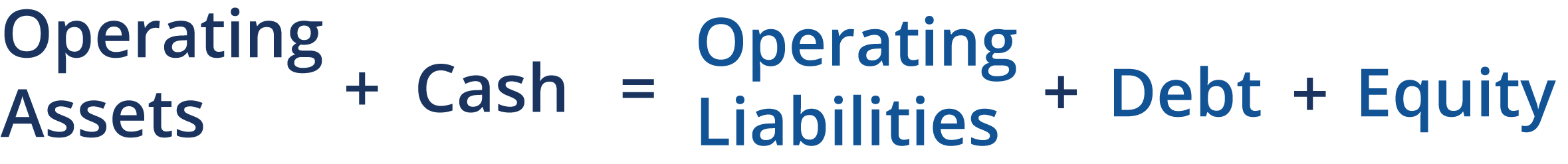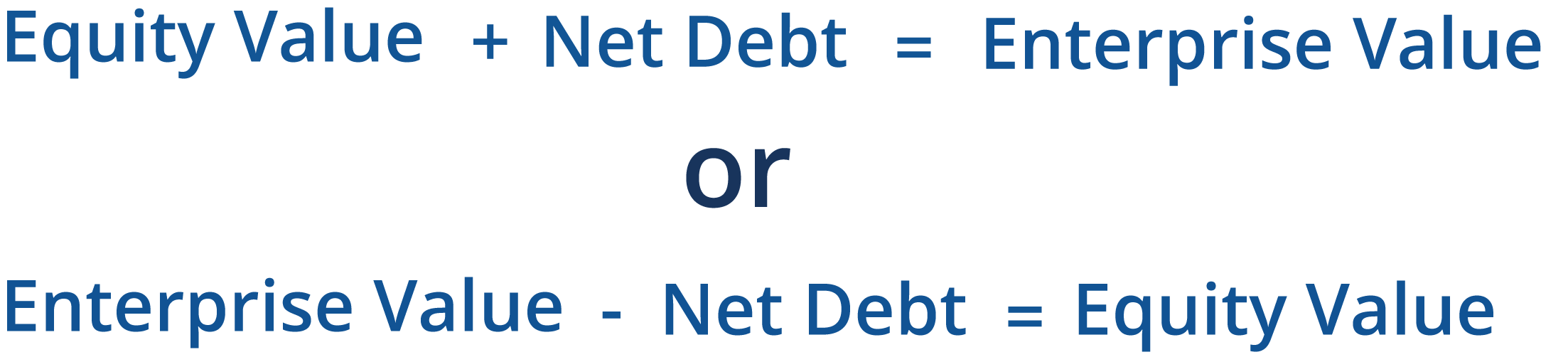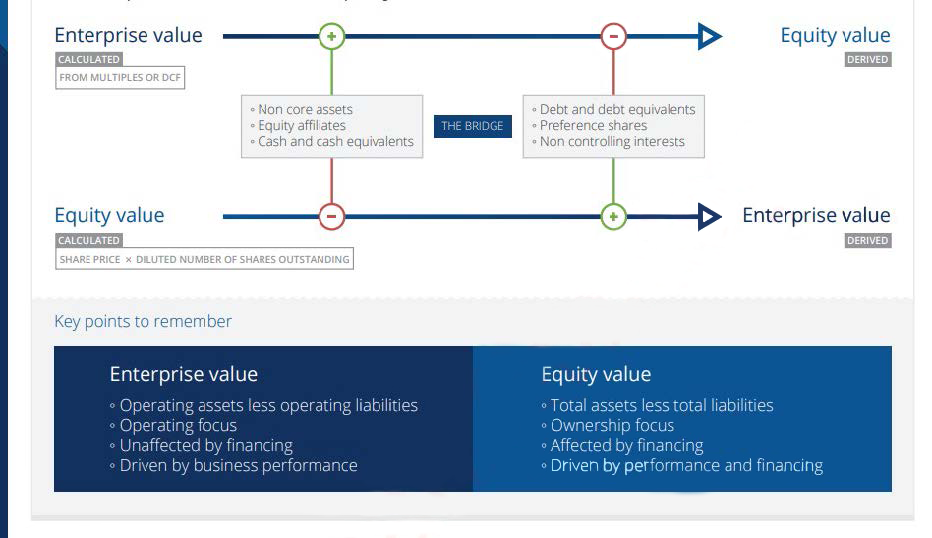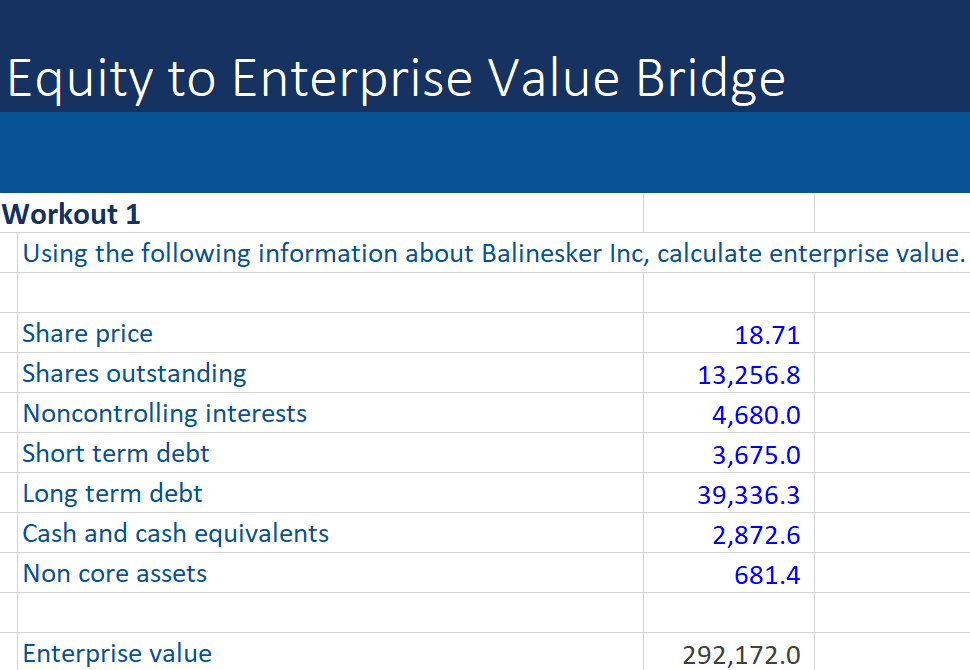## What is the EV to Equity Bridge?

In corporate finance, the most common types of value are equity and enterprise value. Enterprise value is the value of the operational business, independent of capital structure. Equity value (or market capitalization) is the value attributable to the owners or shareholders (frequently expressed on a per-share basis for public companies).Since enterprise value is the fair value of the net operational assets, the equation can be expanded to identify these components as follows:If the equation is rearranged, the operating components can be isolated:Balance sheet amounts are book or carrying value but for valuation, all components must be at the market or fair value. We assume that cash is excess cash and part of the capital structure.

## Key Learning Points

• The EV to equity bridge explains the relationship between the enterprise value and equity value of a company and is used in trading comparables valuation.
• Enterprise value represents the market value of net operational assets of a business and can be calculated using a discounted cash flow analysis.
• Equity value is also known as market capitalization and is calculated as the current share price multiplied by the diluted number of shares. It is the value attributable to the common shareholders of the business.
• There are many items to be considered in the bridge but the general formula is Enterprise Value (EV) equals net debt plus equity.

## Summarizing the EV to Equity Value Bridge

The relationship between enterprise and equity value can be summarized as follows:Since enterprise value equals net debt plus equity value, enterprise value can be derived from equity value and vice versa. In trading comparables, for example, the starting point is the calculation of equity value and from this enterprise value is derived. A discounted cash flow valuation, on the other hand, calculates enterprise value from which equity value is derived. This is summarized below:Download the free Excel Template to see the solution and test it yourself.